# Consider the following information on a portfolio of three stocks: State of Economy Probability of State of Economy Stock A Rate of Return Stock B Rate of Return Stock C Rate of Return Boom .15 ....

Consider the following information on a portfolio of three stocks:

 State of Economy Probability of State of Economy Stock A Rate of Return Stock B Rate of Return Stock C Rate of Return Boom .15 .05 .21 .18 Normal .80 .08 .15 .07 Recession .05 .12 -.22 -.02

The portfolio is invested 35 percent in each Stock A and Stock B and 30 percent in Stock C. If the expected T-bill rate is 3.90 percent, what is the expected risk premium on the portfolio? What is the standard deviation of the portfolio?

E(r) =[Pi x Ri]

E(rA) = [0.15 x 5%] + [0.80 x 8%] + [0.05 x 12%] = 0.75% + 6.40% + 0.60% = 7.75%

E(rB) = [0.15 x 21%] + [0.80 x 15%] + [0.05 x -22%] = 3.15% + 12.00% - 1.10% = 14.05%

E(rC) = [0.15 x 18%] + [0.80 x 7%] + [0.05 x -2%] = 2.70% + 5.60% - 0.10% = 8.20%

E(rP) = [0.35 x 7.75%] + [0.35 x 14.05%] + [0.30 x 8.20%] = 2.71% + 4.92% + 2.46% = 10.09%

E(RP) = E(rP) - rF = 10.09% - 3.90% = 6.19%P = [{Pi x (E(rP) - Ri)2}]1/2

= [{0.35 x (10.09% - 7.75%)2} + {0.35 x (10.09% - 14.05%)2} + {0.30 x (10.09% - 8.20%)2}]1/2

= [1.92%2 + 5.49%2 + 1.07%2]1/2 = [8.48%2]1/2 = 2.91%

##### Add Answer of: Consider the following information on a portfolio of three stocks: State of Economy Probability of State of Economy Stock A Rate of Return Stock B Rate of Return Stock C Rate of Return Boom .15 ....
Similar Homework Help Questions
• ### Consider the following information on a portfolio of three stocks: State of Economy Probability of State...

Consider the following information on a portfolio of three stocks: State of Economy Probability of State of Economy Stock A Rate of Return Stock B Rate of Return Stock C Rate of Return   Boom .13 .10 .35 .42   Normal .52 .18 .30 .28   Bust .35 .19 –.29 –.38 Required: (a) If your portfolio is invested 42 percent each in A and B and 16 percent in C, what is the portfolio’s expected return, the variance, and the standard deviation? (Do...

• ### Consider the following information on a portfolio of three stocks: State of of Economy " Probability of Economy .15...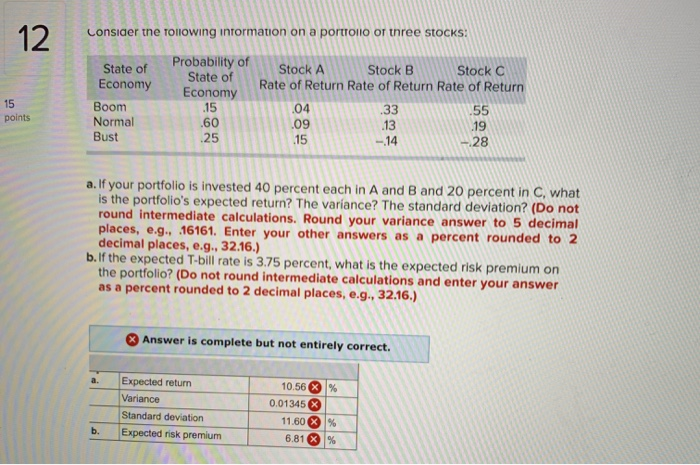Consider the following information on a portfolio of three stocks: State of of Economy " Probability of Economy .15 State of 15 points Boom Normal Bust Stock A Stock B 1 Stock Rate of Return Rate of Return Rate of Return .04 .33 -55 .09 .15 -14 - 28 .19 25 a. If your portfolio is invested 40 percent each in A and B and 20 percent in C, what is the portfolio's expected return? The variance? The standard deviation?...

• ### Consider the following information: State of Economy Boom Bust Probability of State of Economy .70 Rate...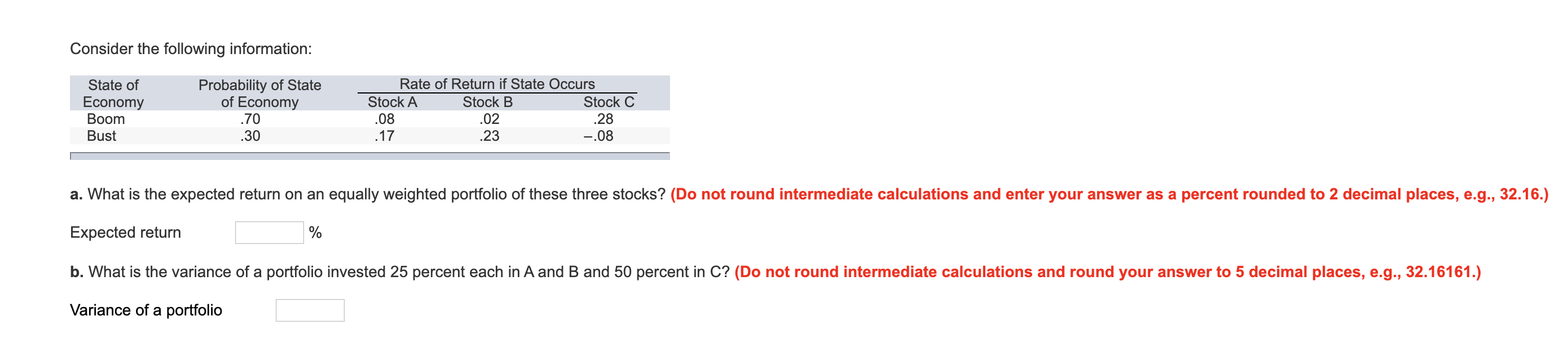Consider the following information: State of Economy Boom Bust Probability of State of Economy .70 Rate of Return if State Occurs Stock A Stock B Stock C 02 .28 .17 .08 .30 .23 -.08 a. What is the expected return on an equally weighted portfolio of these three stocks? (Do not round intermediate calculations and enter your answer as a percent rounded to 2 decimal places, e.g., 32.16.) Expected return b. What is the variance of a portfolio invested 25...

• ### Consider the following information on a portfolio of three stocks Probability of State of State of...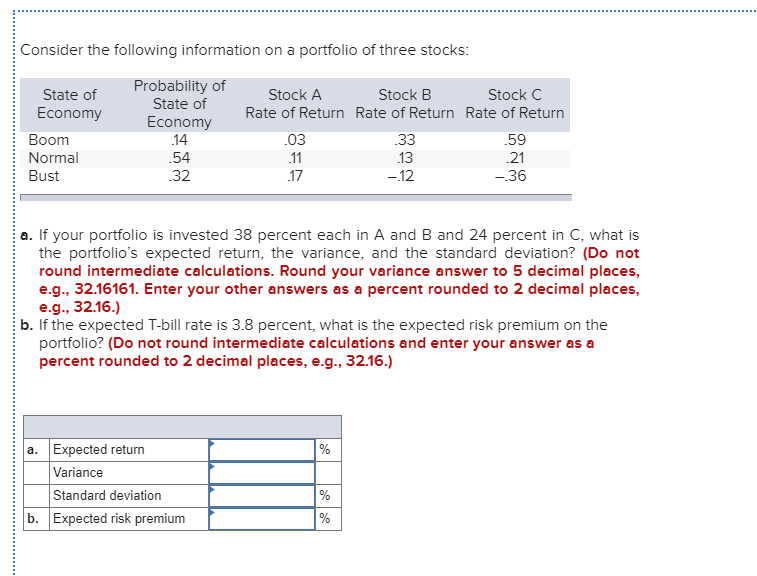Consider the following information on a portfolio of three stocks Probability of State of State of Stock A Stock B Stock C Economy Rate of Return Rate of Return Rate of Return Economy 14 Boom 03 .33 .59 Normal 54 11 13 21 Bust .32 17 -12 -36 a. If your portfolio is invested 38 percent each in A and B and 24 percent in C, what is the portfolio's expected return, the variance, and the standard deviation? (Do not...

• ### Consider the following information on a portfolio of three stocks: TTT Probability of State of State...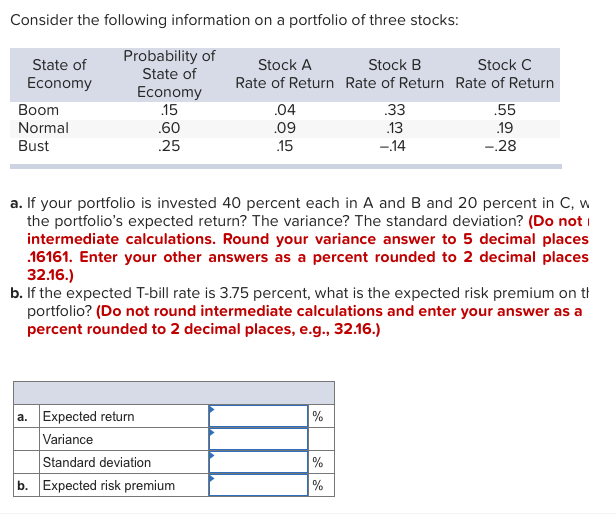Consider the following information on a portfolio of three stocks: TTT Probability of State of State of Stock A Stock B Stock C Economy Rate of Return Rate of Return Rate of Return Economy 15 Вoom 04 .33 .55 Normal 60 09 13 19 Bust 25 15 -14 -28 a. If your portfolio is invested 40 percent each in A and B and 20 percent in C, w the portfolio's expected return? The variance? The standard deviation? (Do not intermediate...

• ### Consider the following information: Rate of Return if State Occurs State of Probability of State Economy of Economy Stoc...

Consider the following information: Rate of Return if State Occurs State of Probability of State Economy of Economy Stock A Stock B Stock C Boom .75 .07 .01 .27 Bust .25 .12 .19 –.05 Required: (a) What is the expected return on an equally weighted portfolio of these three stocks? (Do not round intermediate calculations. Enter your answer as a percentage rounded to 2 decimal places (e.g., 32.16).) Expected return % (b) What is the variance of a portfolio invested...

• ### Consider the following information about three stocks: Rate of Return If State Occurs State of Probability of Economy Economy Boom Normal Bust State of Stock B 56 .14 -.46 25 45 .30 25 .22 .30 .30 c-...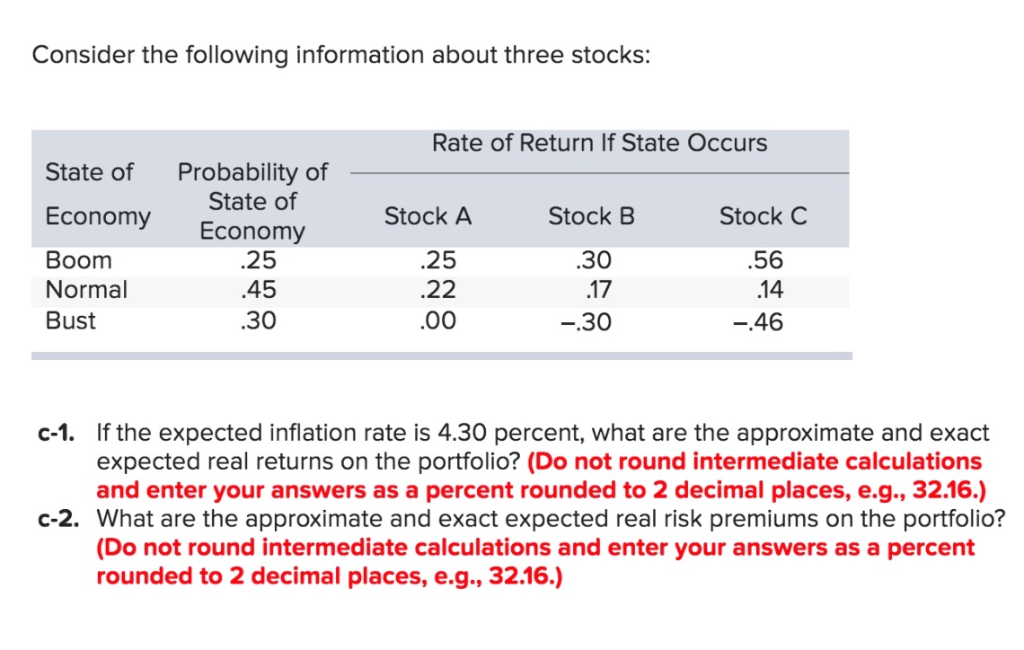Consider the following information about three stocks: Rate of Return If State Occurs State of Probability of Economy Economy Boom Normal Bust State of Stock B 56 .14 -.46 25 45 .30 25 .22 .30 .30 c-1. If the expected inflation rate is 4.30 percent, what are the approximate and exact expected real returns on the portfolio? (Do not round intermediate calculations and enter your answers as a percent rounded to 2 decimal places, e.g., 32.16.) c-2. What are the...

• ### Consider the following information about three stocks: Rate of Return If State Occurs State of Probability...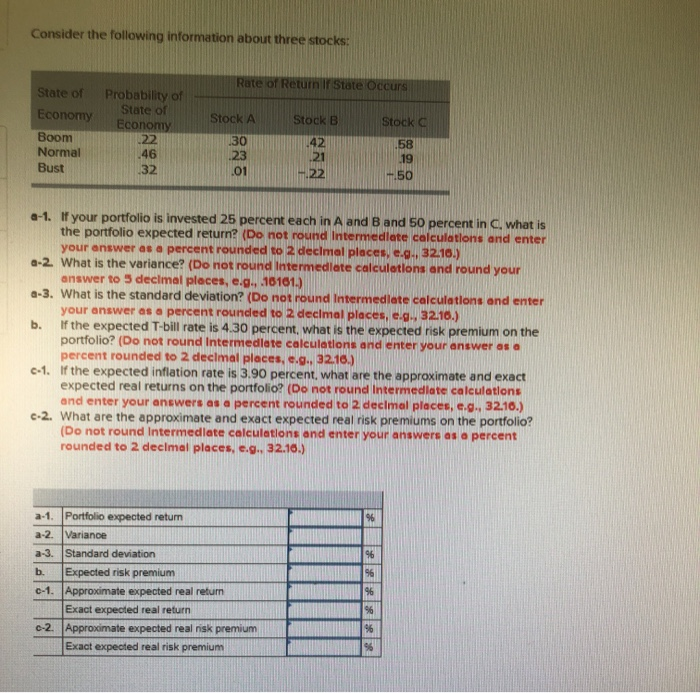Consider the following information about three stocks: Rate of Return If State Occurs State of Probability of State of Economy 22 Economy Stock A Stock B Stock C Boom 30 23 42 21 58 19 Normal 46 Bust 32 01 .22 50 a-1. If your portfolio is invested 25 percent each in A and B and 50 percent in C, what is he portfolio expected return? (Do not round Intermedlate calculations and enter your answer as a percent rounded to...

• ### Consider the following information: Rate of Return If State Occurs State of Economy Boom Probability of...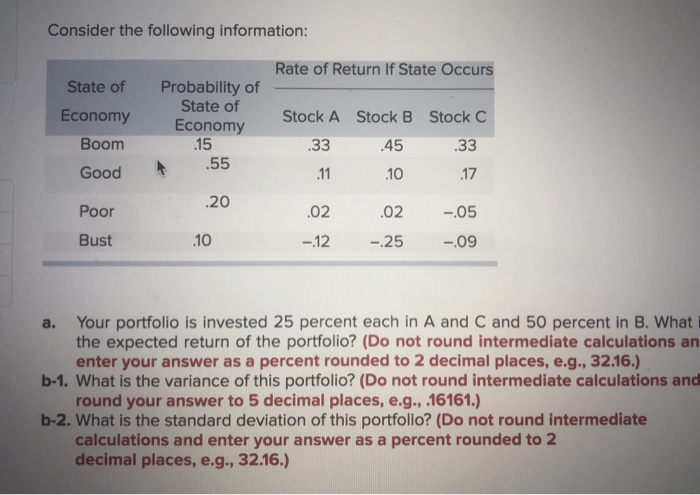Consider the following information: Rate of Return If State Occurs State of Economy Boom Probability of State of Economy .15 Good .55 Stock A .33 11 .02 -12 Stock B 45 10 .02 -.25 Stock C 33 17 -05 -09 .20 Poor Bust 10 a. Your portfolio is invested 25 percent each in A and C and 50 percent in B. What the expected return of the portfolio? (Do not round intermediate calculations an enter your answer as a percent...

• ### Given the following information, What is the expected return on a portfolio which is invested 40% in Stock X and 60% in Stock Y? State of Economy Prob. Stock X Stock Y Boom 10% 25% -17% Normal...

Given the following information, What is the expected return on a portfolio which is invested 40% in Stock X and 60% in Stock Y? State of Economy Prob. Stock X Stock Y Boom 10% 25% -17% Normal 70% 10% 7% Recession 20% -15% 30%

Need Online Homework Help?